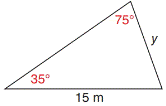Chapter 11.4, Problem 19E### Elementary Geometry for College St...

6th Edition
Daniel C. Alexander + 1 other
ISBN: 9781285195698

#### Solutions

Chapter
Section### Elementary Geometry for College St...

6th Edition
Daniel C. Alexander + 1 other
ISBN: 9781285195698
Textbook Problem
1 views

# In Exercises 17 to 22, use a form of the Law of Sines to find the measure of the indicated side or angle. Angle measures should be found to the nearest degree and lengths of sides to the nearest tenth of a unit.To determine

To find:

The measure of the side in given triangle, by using the Law of Sines,

Explanation

Formula:

The Law of Sines,

sinαa=sinβb=sinγc

Where α, β, and γ is the acute angle of the triangle, and a, b, and c is the sides of the triangle.

Calculation:

Given,

From the figure,

β=75°

γ=35°

b=15 m.

c=y

Find y:

By the Law of Sines,

sinαa=sinβb=sinγc

Take,

sinαa=sinγc

Substitute b=15 m, c=y m,<

### Still sussing out bartleby?

Check out a sample textbook solution.

See a sample solution

#### The Solution to Your Study Problems

Bartleby provides explanations to thousands of textbook problems written by our experts, many with advanced degrees!

Get Started

#### Find more solutions based on key concepts